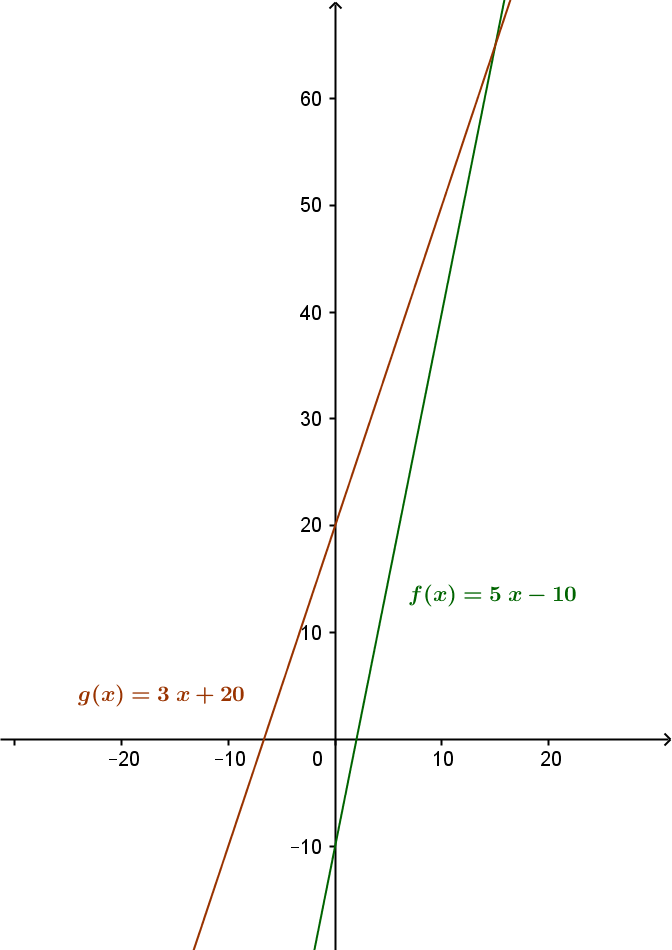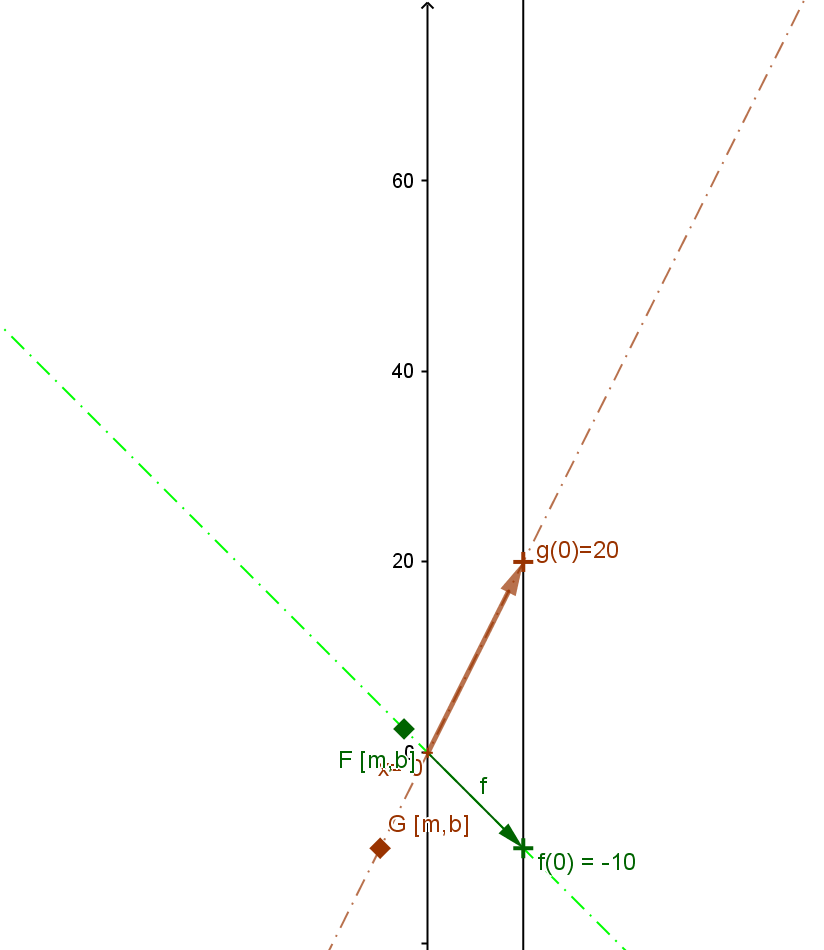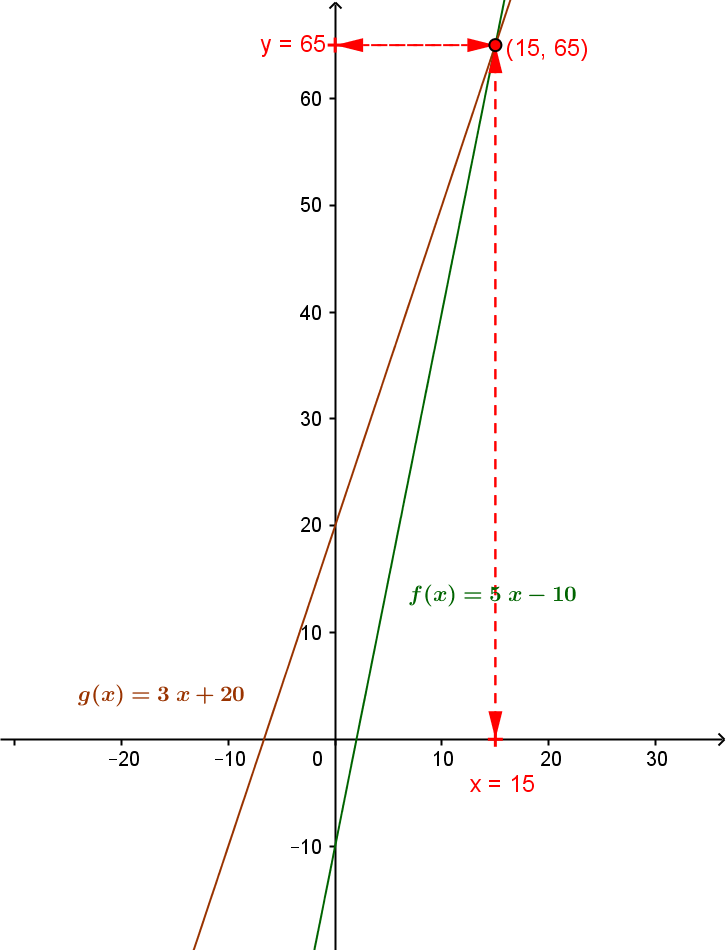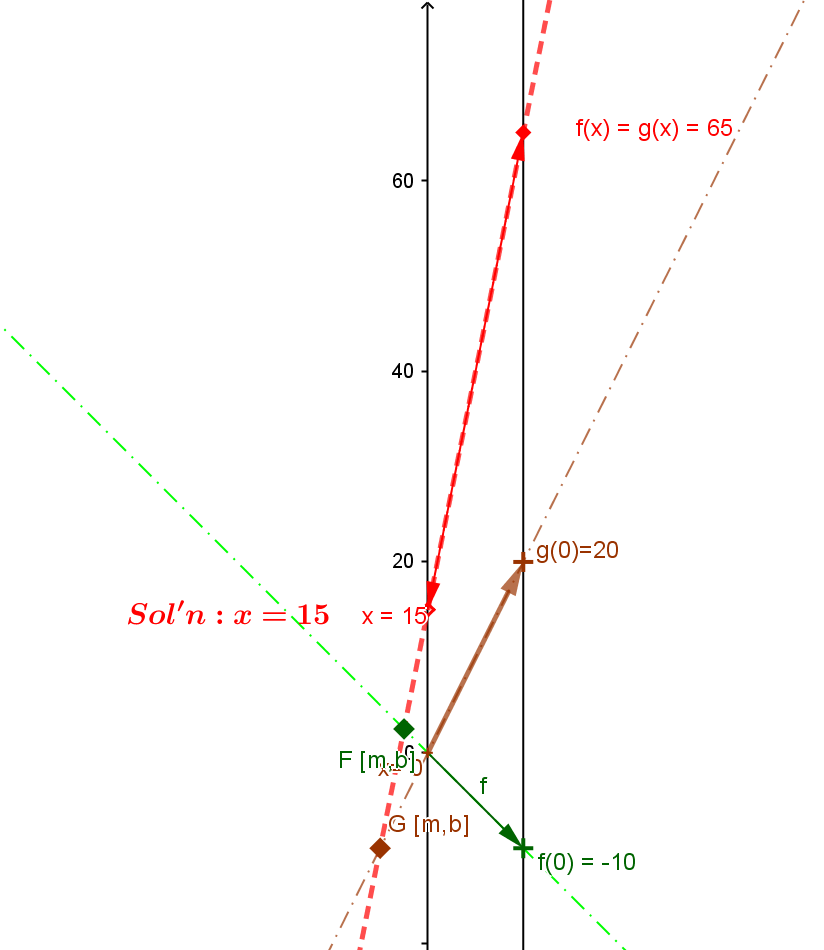Example  2.  Suppose $5x - 10 = 3x + 20$. Find $x$.
Solution: Since $A_1 \ne A_2$, the equation can be solved so that $x = \frac {C- B} A$  where $A=5-3=2, B = -10$, and $C = 20$. So the solution is $x = \frac {20 - (-10)} 2 = \frac {30} 2 = 15.$

Comment: We can consider the expression on the left hand side of the equation as a linear function of $x$ giving $f(x) = 5x - 10$ . The expression on the right side of the equation can also be considered as a linear function of $x$, $g(x) = 3x +20$.
The problem can be restated now: To find a value for $x$ where $f(x) = g(x)$.
This problem and its solution can be visualized both on the graphs and the mapping diagrams with focus points for the linear functions $f$ and $g$.
 For the graphs of $f$ and $g$: Find the first coordinate for the point on the graph of $f$ which is also on the graph of $g$. For the mapping diagrams of $f$ and $g$: Find $x$ on the source axis with the function arrow for $f$ coinciding with the function arow for $g$.To do this, look for the point where the lines that graph $g$  and $f$ intersect. The first coordinate of that point, $15$, is the desired value of $x$. To do this,  find the focus point of $f$, $F= [5, -10]$ on the mapping diagram. and the focus point of $g$, $G=[3,20]$ .[Use Geogebra?] Draw the line through F and G to find the point of intersection of this line with the X axis, $x=15$, which is the desired value for $x$.